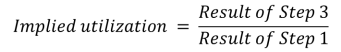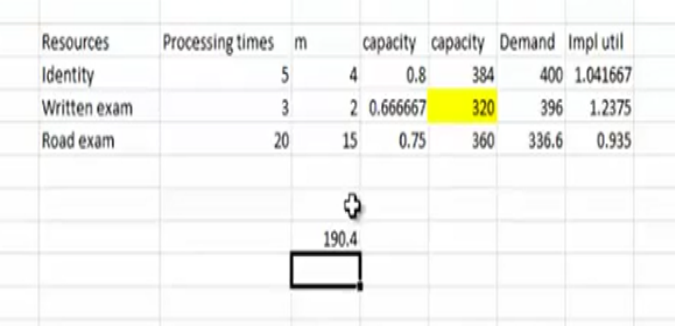×

×

PENN / Operations,Information & Strategic Mgmt / OIDD 220 / What is the meaning of flow rate/throughput?

# What is the meaning of flow rate/throughput? Description

##### Description: These are my notes for Module 1 - An Introduction to Operations Management - at Wharton taught by Christian Terwiesch. They are a summary of ideas and concepts for the course, which has been super helpful for me, and will hopefully be helpful for you too! Let me know how you like them...any feedback is appreciated.
14 Pages 86 Views 11 Unlocks
Reviews

Mario Viti? (Rating: )

Summary

## What is the meaning of flow rate/throughput?When observing a process, always aim to understand the three process measures  • Flow rate / throughput: number of flow units going through the process per unit of =me  • Flow Time: =me it takes a flow unit to go from the beginning to the end of the process  • Inventory: the number of flow units in the process at a given moment in =me  In the next session, we will discuss what drives these measures

We will then find out that the three measures are related to each other

Basic Process Vocabulary

• Processing 9mes: how long does the worker spend on the task?

• Capacity=1/processing =me: how many units can the worker make per unit of =me     If there are m workers at the ac=vity: Capacity=m/ac=vity =me

## What is the meaning of flow time?If you want to learn more check out What is the meaning of eternal energy?
Don't forget about the age old question of How much has group size changed?

• Bo<leneck: process step with the lowest capacity  Don't forget about the age old question of Who are tecumseh and tenskwatawa?
We also discuss several other topics like Columbus was born when?

• Process capacity: capacity of the boJleneck

• Flow rate =Minimum{Demand rate, Process Capacity)

• U9liza9on =Flow Rate / Capacity

• Flow Time: The amount of =me it takes a flow unit to go through the process  • Inventory: The number of flow units in the system

Labor Produc=vity Measures  BoJleneck

e

m

i

T

a4 a2

=Idle Time

=Processing =me

## What is the meaning of processing times?g

n

is

s

e

c

o

r

P

a1

a3

1  2  3  4

Review of Capacity Calcula9ons

Labor Produc9vity Measures

•  Cycle =me CT= 1/ Flow Rate

Direct Labor Content=p1+p2+p3+p4   If one worker per resource:  If you want to learn more check out Who is huckabee?

Direct Idle Time=(CT-p1) +(CT-p2) +(CT-p3)

• Average labor u<liza<on

labor content

+ =

• Capacityi  =

Number of Resourcesilabor content direct idle time

Processing Timei If you want to learn more check out What are the characteristics of the solar system?

• Process Capacity=Min{Capacityi}  • Flow Rate = Min{Demand, Capacity}

• Cost of direct labor

Total wages per unit of time = Flow Rate per unit of time

• U<liza<oni=

Flow Rate Capacityi

LiJle’s law: It’s more powerful than  you think...

What it is:        Inventory (I) = Flow Rate (R) * Flow Time (T)

How to remember it: - units

Implica9ons:

• Out of the three fundamental performance measures (I,R,T), two can be chosen by     management, the other is GIVEN by nature

• Hold throughput constant: Reducing inventory = reducing flow =me

Given two of the three measures, you can solve for the third:

• Indirect measurement of flow =me: how long does it take you on average to respond to an email?     You write 60 email responses per day

You have 240 emails in your inbox

Steps for Basic Process Analysis with  Mul=ple Types of Flow Units

1. For each resource, compute the number of minutes that the resource can  produce

2. Create a process flow diagram, indica=ng how the flow units go through the  process

3. Create a table indica=ng how much workload each flow unit is consuming at  each resource

4. Add up the workload of each resource across all flow units.

5. Compute the implied u=liza=on of each resource asThe resource with the highest implied u=liza=on is the boJleneck

Note: you can also find the boJleneck based on calcula=ng capacity for each step and  then dividing the demand at this resource by the capacity

m/PT

4/5=0.8

Cap/min  *60mins* 8hr/dayNo will get licence = 320 * 0.85 * 0.70

Page ExpiredIt looks like your free minutes have expired! Lucky for you we have all the content you need, just sign up here